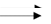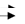﻿ (Rod Mill-Ball Mill Circuit) or (SAG Mill-Ball Mill Circuit)
Ball Mill Design Calculations
(Rod Mill-Ball Mill Circuit) or (SAG Mill-Ball Mill Circuit)

Model Input     Model Output
Feed rate to mill MTPH     Total work required to produce the product (kwh/short ton)
Feed rate to mill STPH     Total kW Required
Feed F80 (μm)     Total Power Required (HP)
Product P80 (μm)     Power Required/Ball Mill (HP)
Work index Kwh/short tonEF3 Diameter efficiency (Table IX & VII) (Review Mill Diameter VS Horse Power)
Power required per ball mill (updated), HPNumber of Ball Mills   1) Suggested Ball Mill Diameter (1st iteration) Table VII
1.5 Suggested L/D Ratio (Table VI)
2) Suggested Ball Mill HP (1st iteration) Table VII
3) L, Suggested Ball Mill Length (1st iteration) Table VII

Ratio: Needed HP/Suggested Ball Mill HP
L, Required Ball Mill Length (m)
**Select Nearest Mill with 40% Charge

Use Ball Mill meters Long X meter in Diameter Overflow Discharge
Using Table VII a Grate Discharge Ball Mill Can by Sized by selecting 1) 2) 3)

Disclaimer
-